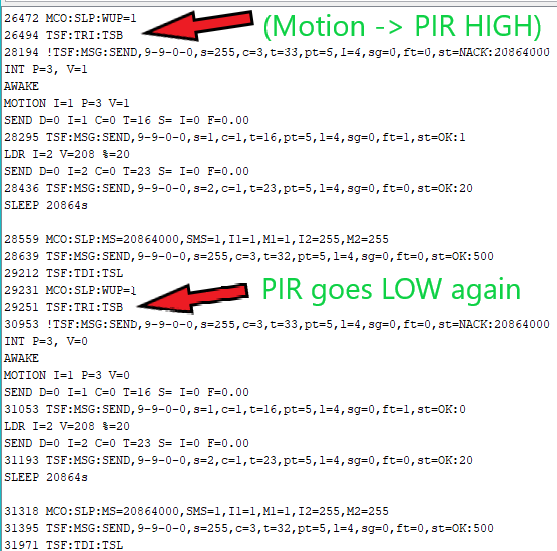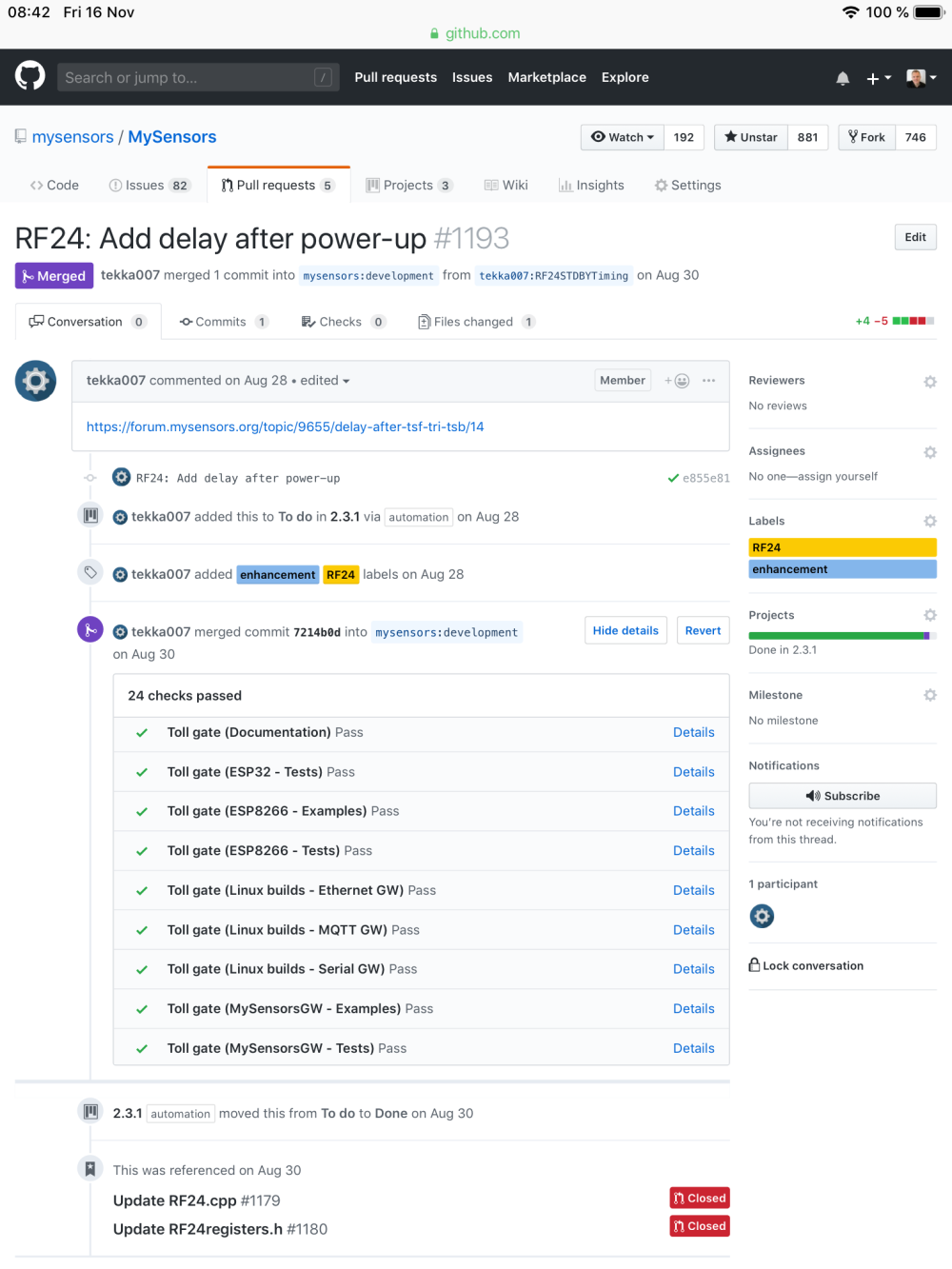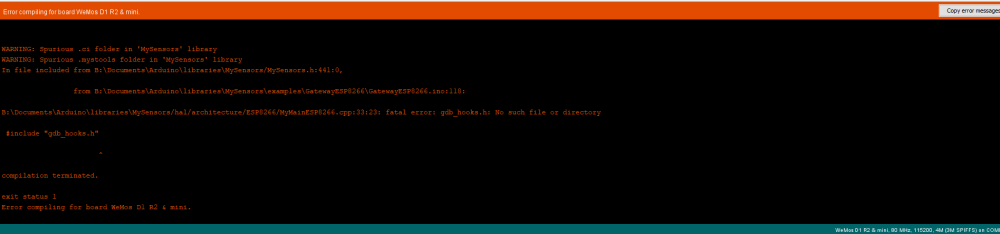# Delay after TSF:TRI:TSB

• Hi I’ve build an Motion+Light Sensor (connected to openHab) and it works pretty well, except that there is a tiny delay (1-2 sec) between waking up and sending the message – which is a bit of a pain, since e.g. connected lights will turn on with an delay.

What I found out is, that the reason for the delay lies in the sensor itself – so no problems with the gateway. In the DEBUG of the sensor you can see TSF:TRI:TSB, and right there the sensor stops for about 1-2 seconds before sending it’s msg. (see picture below):Do I need to change somthing in "MyTransport" in order to get rid of this delay -> “TSB “ (Set transport to standby)? https://www.mysensors.org/apidocs-beta/group__MyTransportgrp.html#ga2c5750b886bd43338e04cd4cb132e589

Or is there any other way - maybe I'm missing something (sketch down below).

Hope You can help - many thanks in advance``````/*
[...]
/**********************************
* MySensors node configuration
*/

// General settings
#define SKETCH_NAME "Testo"
#define SKETCH_VERSION "2.0"
#define MY_DEBUG

//#define MY_SIGNING_SIMPLE_PASSWD 1234
#define MY_RF24_PA_LEVEL RF24_PA_HIGH

#define MY_BAUD_RATE 9600
#define MY_SPLASH_SCREEN_DISABLED

/***********************************
* NodeManager modules for supported sensors
*/

#define USE_ANALOG_INPUT
#define USE_INTERRUPT

/***********************************
* NodeManager built-in features
*/

// Enable/disable NodeManager's features
#define FEATURE_DEBUG ON
#define FEATURE_POWER_MANAGER ON
#define FEATURE_INTERRUPTS ON
#define FEATURE_CONDITIONAL_REPORT OFF
#define FEATURE_EEPROM OFF
#define FEATURE_SLEEP ON
#define FEATURE_TIME OFF
#define FEATURE_RTC OFF
#define FEATURE_SD OFF
#define FEATURE_HOOKING OFF

/***********************************
*/

#include "NodeManagerLibrary.h"
NodeManager node;

/***********************************
*/

// built-in sensors

PowerManager power(5,6,0);

// Attached sensors

SensorMotion motion(node,3);
SensorLDR ldr(node,A0);

/***********************************
* Main Sketch
*/

// before
void before() {
// setup the serial port baud rate
Serial.begin(MY_BAUD_RATE);
/*
*/
node.setReportIntervalSeconds(60);
node.setSleepDays(1);
/*
*/
node.before();
}

// presentation
void presentation() {
// call NodeManager presentation routine
node.presentation();
}

// setup
void setup() {
// call NodeManager setup routine
node.setup();
}

// loop
void loop() {
// call NodeManager loop routine
node.loop();
}

}
#endif

#if FEATURE_TIME == ON
}
#endif
``````

• @fausti the line after indicated NACK. This means that the node did not get acknowledgement from the next node (usually the gateway). The node can't print the nack message until after it has tried and gotten timeout several times. That's why it takes some time. The first send attempt starts much earlier.

• @mfalkvidd many thanks for the quick answer!Weirdly this behaviour only shows when I'm using the sleep mode. Also first time NACK appears is after the first Sleep - DEBUG after reset below, NACK at 8437. Any idea what i'm doing wrong or where i could fix it?many thanks in advance``````⸮:⸮5 V=6
0 MCO:BGN:INIT NODE,CP=RNNNA---,VER=2.3.0
53 MCO:BGN:BFR
NodeManager v1.7
LIB V=2.3.0 R=N E=- T=N A=A S=- B=-
MOTION I=1 P=1 T=16
LDR I=2 P=16 T=23
190 TSF:WUR:MS=0
215 TSM:INIT:TSP OK
235 TSF:SID:OK,ID=9
256 TSM:FPAR
305 TSF:MSG:SEND,9-9-255-255,s=255,c=3,t=7,pt=0,l=0,sg=0,ft=0,st=OK:
2402 TSM:FPAR
2451 TSF:MSG:SEND,9-9-255-255,s=255,c=3,t=7,pt=0,l=0,sg=0,ft=0,st=OK:
3334 TSF:MSG:FPAR OK,ID=0,D=1
4526 TSM:FPAR:OK
4542 TSM:ID
4556 TSM:ID:OK
4571 TSM:UPL
4589 TSF:MSG:SEND,9-9-0-0,s=255,c=3,t=24,pt=1,l=1,sg=0,ft=0,st=OK:1
4718 TSF:MSG:PONG RECV,HP=1
4747 TSM:UPL:OK
4802 TSF:MSG:SEND,9-9-0-0,s=255,c=3,t=15,pt=6,l=2,sg=0,ft=0,st=OK:0100
4941 TSF:MSG:SEND,9-9-0-0,s=255,c=0,t=17,pt=0,l=5,sg=0,ft=0,st=OK:2.3.0
5021 TSF:MSG:SEND,9-9-0-0,s=255,c=3,t=6,pt=1,l=1,sg=0,ft=0,st=OK:0
OK
5152 TSF:MSG:SEND,9-9-0-0,s=255,c=3,t=11,pt=0,l=5,sg=0,ft=0,st=OK:Testo
5232 TSF:MSG:SEND,9-9-0-0,s=255,c=3,t=12,pt=0,l=3,sg=0,ft=0,st=OK:2.0
PRES I=1 T=1 D=MOTION
5310 TSF:MSG:SEND,9-9-0-0,s=1,c=0,t=1,pt=0,l=6,sg=0,ft=0,st=OK:MOTION
PRES I=2 T=16 D=LDR
5406 TSF:MSG:SEND,9-9-0-0,s=2,c=0,t=16,pt=0,l=3,sg=0,ft=0,st=OK:LDR

5494 MCO:REG:REQ
5525 TSF:MSG:SEND,9-9-0-0,s=255,c=3,t=26,pt=1,l=1,sg=0,ft=0,st=OK:2
5656 TSF:MSG:ACK
5732 TSF:MSG:ACK
5808 MCO:PIM:NODE REG=1
5832 MCO:BGN:STP
MY I=9 M=1
INT P=3 M=1
INT P=2 M=255
5853 MCO:BGN:INIT OK,TSP=1
LDR I=2 V=60 %=5
SEND D=0 I=2 C=0 T=23 S= I=0 F=0.00
5928 TSF:MSG:SEND,9-9-0-0,s=2,c=1,t=23,pt=5,l=4,sg=0,ft=0,st=OK:5
SLEEP 20864s

6047 MCO:SLP:MS=20864000,SMS=1,I1=1,M1=1,I2=255,M2=255
6125 TSF:MSG:SEND,9-9-0-0,s=255,c=3,t=32,pt=5,l=4,sg=0,ft=0,st=OK:500
6254 TSF:MSG:ACK
6699 TSF:TDI:TSL
6717 MCO:SLP:WUP=1
6735 TSF:TRI:TSB
8437 !TSF:MSG:SEND,9-9-0-0,s=255,c=3,t=33,pt=5,l=4,sg=0,ft=0,st=NACK:20864000
INT P=3, V=1
AWAKE
MOTION I=1 P=3 V=1
SEND D=0 I=1 C=0 T=16 S= I=0 F=0.00
8536 TSF:MSG:SEND,9-9-0-0,s=1,c=1,t=16,pt=5,l=4,sg=0,ft=1,st=OK:1
LDR I=2 V=59 %=5
SEND D=0 I=2 C=0 T=23 S= I=0 F=0.00
8675 TSF:MSG:SEND,9-9-0-0,s=2,c=1,t=23,pt=5,l=4,sg=0,ft=0,st=OK:5
SLEEP 20864s

8796 MCO:SLP:MS=20864000,SMS=1,I1=1,M1=1,I2=255,M2=255
8869 TSF:MSG:SEND,9-9-0-0,s=255,c=3,t=32,pt=5,l=4,sg=0,ft=0,st=OK:500
8998 TSF:MSG:ACK
9072 TSF:MSG:ACK
9443 TSF:TDI:TSL
9459 MCO:SLP:WUP=1
9480 TSF:TRI:TSB
11180 !TSF:MSG:SEND,9-9-0-0,s=255,c=3,t=33,pt=5,l=4,sg=0,ft=0,st=NACK:20864000
INT P=3, V=0
AWAKE
MOTION I=1 P=3 V=0
SEND D=0 I=1 C=0 T=16 S= I=0 F=0.00
11280 TSF:MSG:SEND,9-9-0-0,s=1,c=1,t=16,pt=5,l=4,sg=0,ft=1,st=OK:0
LDR I=2 V=60 %=5
SEND D=0 I=2 C=0 T=23 S= I=0 F=0.00
11421 TSF:MSG:SEND,9-9-0-0,s=2,c=1,t=23,pt=5,l=4,sg=0,ft=0,st=OK:5
SLEEP 20864s

11542 MCO:SLP:MS=20864000,SMS=1,I1=1,M1=1,I2=255,M2=255
11620 TSF:MSG:SEND,9-9-0-0,s=255,c=3,t=32,pt=5,l=4,sg=0,ft=0,st=OK:500
11751 TSF:MSG:ACK
11827 TSF:MSG:ACK
12197 TSF:TDI:TSL
``````

Here the DEBUG if I exclude sleep (to --> //node.setSleepDays(1);) - then the sensor sends it's msg. fast and happily..

``````⸮:⸮5 V=6
0 MCO:BGN:INIT NODE,CP=RNNNA---,VER=2.3.0
53 MCO:BGN:BFR
NodeManager v1.7
LIB V=2.3.0 R=N E=- T=N A=A S=- B=-
MOTION I=1 P=1 T=16
LDR I=2 P=16 T=23
190 TSF:WUR:MS=0
215 TSM:INIT:TSP OK
235 TSF:SID:OK,ID=9
256 TSM:FPAR
305 TSF:MSG:SEND,9-9-255-255,s=255,c=3,t=7,pt=0,l=0,sg=0,ft=0,st=OK:
432 TSF:MSG:FPAR OK,ID=0,D=1
2377 TSM:FPAR:OK
2394 TSM:ID
2408 TSM:ID:OK
2422 TSM:UPL
2441 TSF:MSG:SEND,9-9-0-0,s=255,c=3,t=24,pt=1,l=1,sg=0,ft=0,st=OK:1
2570 TSF:MSG:PONG RECV,HP=1
2598 TSM:UPL:OK
2654 TSF:MSG:SEND,9-9-0-0,s=255,c=3,t=15,pt=6,l=2,sg=0,ft=0,st=OK:0100
2793 TSF:MSG:SEND,9-9-0-0,s=255,c=0,t=17,pt=0,l=5,sg=0,ft=0,st=OK:2.3.0
2873 TSF:MSG:SEND,9-9-0-0,s=255,c=3,t=6,pt=1,l=1,sg=0,ft=0,st=OK:0
OK
3035 TSF:MSG:SEND,9-9-0-0,s=255,c=3,t=11,pt=0,l=5,sg=0,ft=0,st=OK:Testo
3112 TSF:MSG:SEND,9-9-0-0,s=255,c=3,t=12,pt=0,l=3,sg=0,ft=0,st=OK:2.0
PRES I=1 T=1 D=MOTION
3190 TSF:MSG:SEND,9-9-0-0,s=1,c=0,t=1,pt=0,l=6,sg=0,ft=0,st=OK:MOTION
PRES I=2 T=16 D=LDR
3289 TSF:MSG:SEND,9-9-0-0,s=2,c=0,t=16,pt=0,l=3,sg=0,ft=0,st=OK:LDR

3377 MCO:REG:REQ
3407 TSF:MSG:SEND,9-9-0-0,s=255,c=3,t=26,pt=1,l=1,sg=0,ft=0,st=OK:2
3536 MCO:PIM:NODE REG=1
3561 MCO:BGN:STP
MY I=9 M=1
INT P=3 M=1
INT P=2 M=255
3581 MCO:BGN:INIT OK,TSP=1
LDR I=2 V=57 %=5
SEND D=0 I=2 C=0 T=23 S= I=0 F=0.00
3657 TSF:MSG:SEND,9-9-0-0,s=2,c=1,t=23,pt=5,l=4,sg=0,ft=0,st=OK:5
INT P=3, V=1
MOTION I=1 P=3 V=1
SEND D=0 I=1 C=0 T=16 S= I=0 F=0.00
8374 TSF:MSG:SEND,9-9-0-0,s=1,c=1,t=16,pt=5,l=4,sg=0,ft=0,st=OK:1
INT P=3, V=0
MOTION I=1 P=3 V=0
SEND D=0 I=1 C=0 T=16 S= I=0 F=0.00
12304 TSF:MSG:SEND,9-9-0-0,s=1,c=1,t=16,pt=5,l=4,sg=0,ft=0,st=OK:0
``````

• @fausti sorry, I don't know what nodemanager does behind the scene so there isn't really a way for me to troubleshoot.

What is powered through pin 6?

• @mfalkvidd thanks again!!Yeah.. went through the whole NodeManager Library, tried many things, but couldn’t find the reason for this behaviour. probably I must build my sketch again on foot – without NodeManager, hoped it could save me some time. ^^
PIN 6 powers an LDR together with PIN 5, read by PIN A0. But when I exclude all corresponding parts and even remove the hardware (10k + LDR) it won’t help.

Oddly sleeping Time (MCO:SLP:MS=20864000 has the same value as the number after NACK (st=NACK:20864000). If I change the sleep time (e.g. from 1 day to 60 sec) both values are changing equally to the equivalent value in ms.

@henrik-nielsen had a similar problem (maybe the same) https://forum.mysensors.org/topic/9598/smartsleep-wake-up-message-always-get-nack
Later I’ll try the fix described by @berkseo.

Thanks!• @fausti are you using an amplified nrf24 pa+lna module by any chance?

• @fausti interesting that the sleep and the nack has the same value. Maybe something gets overwritten in memory.

• @mfalkvidd @fausti no, this is normal the smartsleep log:

``````6047 MCO:SLP:MS=20864000,SMS=1,I1=1,M1=1,I2=255,M2=255
``````

==> smart sleep enabled, IRQ on pin 1 set, going to sleep for 20864000 ms

``````6125 TSF:MSG:SEND,9-9-0-0,s=255,c=3,t=32,pt=5,l=4,sg=0,ft=0,st=OK:500
``````

==> I_PRE_SLEEP_NOTIFICATION, 500ms to process any incoming message before going to seep

``````6199 TSF:MSG:READ,0-0-9,s=2,c=1,t=23,pt=5,l=4,sg=0:5
6254 TSF:MSG:ACK
6699 TSF:TDI:TSL
6717 MCO:SLP:WUP=1
6735 TSF:TRI:TSB
``````

==> sleep interrupted by IRQ 1

``````8437 !TSF:MSG:SEND,9-9-0-0,s=255,c=3,t=33,pt=5,l=4,sg=0,ft=0,st=NACK:20864000
``````

==> I_POST_SLEEP_NOTIFICATION, node was sleeping for 20864000ms (but sleep was interrupted)

• ...but, the delay between TSB and SEND is a bit odd;

``````9480 TSF:TRI:TSB
11180 !TSF:MSG:SEND,9-9-0-0,s=255,c=3,t=33,pt=5,l=4,sg=0,ft=0,st=NACK:20864000
``````

Enabling `MY_DEBUG_VERBOSE_RF24` could eventually give some hints.

• FYI: I tried with both, 2.3.0 and 2.3.1-beta (incl. CE fix), on non-amplified and PA+LNA modules - I do not see any delay between TSB and SEND

``````6973 MCO:SLP:MS=5000,SMS=1,I1=1,M1=1,I2=255,M2=255
6979 TSF:MSG:SEND,100-100-0-0,s=255,c=3,t=32,pt=5,l=4,sg=0,ft=0,st=OK:500
7486 TSF:TDI:TSL
7487 MCO:SLP:WUP=1
7489 TSF:TRI:TSB
7492 TSF:MSG:SEND,100-100-0-0,s=255,c=3,t=33,pt=5,l=4,sg=0,ft=0,st=OK:5000
``````

I assume you have a power issue, a bad module - or an artifact originating from the nodemanager?

• @Yveaux sensor is using standard NRF24L01+ (only the gw uses pa+lna - but I guess that won't be an issue?)

@tekka did you use the same sketch? You mentioned smartsleep, I haven't enabled that.. I'll try `MY_DEBUG_VERBOSE_RF24` later, use a fresh NodeManager and will check all my hardware again and I still have to try that fix: https://forum.mysensors.org/topic/9598/smartsleep-wake-up-message-always-get-nack
I've build more than one sensor and all of them are showing that delay - using different power sources and I also tried different capacitor. Maybe after all it's the gw.. but can't think of a reason why sleeping would cause that behavior at gw.

I'll report later - Thanks for the quick help!!

• @fausti the log indicates that smartsleep is enabled - that said, smartsleep may originate from the nodemanager

6047 MCO:SLP:MS=20864000,SMS=1

• @fausti Can you re-test your setup with this patch (ideally, update GW and nodes): https://github.com/tekka007/MySensors/tree/RF24STDBYTiming - I've added a delay after setting the radio in standby mode.

• @tekka WOW!! Your fix works like a dream - can't thank you enough!For now I just used your patch at the sensor - but it allready runs perfectly - no lag/no NACK (see DEBUG below).
So the radio needs more time to settle? How long did you set the delay?

Many many thanks to @tekka and to you all for helping that fast and professional!!

[For so many hours I tried figuring out where the problem lies.. at least I know that library better now ^^ ]

``````0 MCO:BGN:INIT NODE,CP=RNNNA---,VER=2.3.1-beta
59 MCO:BGN:BFR
NodeManager v1.7
LIB V=2.3.1-beta R=N E=- T=N A=A S=- B=-
MOTION I=1 P=1 T=16
LDR I=2 P=16 T=23
200 TSF:WUR:MS=0
223 TSM:INIT:TSP OK
245 TSF:SID:OK,ID=9
266 TSM:FPAR
315 TSF:MSG:SEND,9-9-255-255,s=255,c=3,t=7,pt=0,l=0,sg=0,ft=0,st=OK:
1265 TSF:MSG:FPAR OK,ID=0,D=1
2390 TSM:FPAR:OK
2406 TSM:ID
2420 TSM:ID:OK
2435 TSM:UPL
2453 TSF:MSG:SEND,9-9-0-0,s=255,c=3,t=24,pt=1,l=1,sg=0,ft=0,st=OK:1
2582 TSF:MSG:PONG RECV,HP=1
2611 TSM:UPL:OK
2666 TSF:MSG:SEND,9-9-0-0,s=255,c=3,t=15,pt=6,l=2,sg=0,ft=0,st=OK:0100
2805 TSF:MSG:SEND,9-9-0-0,s=255,c=0,t=17,pt=0,l=10,sg=0,ft=0,st=OK:2.3.1-beta
2891 TSF:MSG:SEND,9-9-0-0,s=255,c=3,t=6,pt=1,l=1,sg=0,ft=0,st=OK:0
OK
3022 TSF:MSG:SEND,9-9-0-0,s=255,c=3,t=11,pt=0,l=5,sg=0,ft=0,st=OK:Testo
3102 TSF:MSG:SEND,9-9-0-0,s=255,c=3,t=12,pt=0,l=3,sg=0,ft=0,st=OK:2.0
PRES I=1 T=1 D=MOTION
3180 TSF:MSG:SEND,9-9-0-0,s=1,c=0,t=1,pt=0,l=6,sg=0,ft=0,st=OK:MOTION
PRES I=2 T=16 D=LDR
3276 TSF:MSG:SEND,9-9-0-0,s=2,c=0,t=16,pt=0,l=3,sg=0,ft=0,st=OK:LDR

3364 MCO:REG:REQ
3395 TSF:MSG:SEND,9-9-0-0,s=255,c=3,t=26,pt=1,l=1,sg=0,ft=0,st=OK:2
3524 MCO:PIM:NODE REG=1
3551 MCO:BGN:STP
MY I=9 M=1
INT P=3 M=1
INT P=2 M=255
3569 MCO:BGN:INIT OK,TSP=1
LDR I=2 V=302 %=29
SEND D=0 I=2 C=0 T=23 S= I=0 F=0.00
3645 TSF:MSG:SEND,9-9-0-0,s=2,c=1,t=23,pt=5,l=4,sg=0,ft=0,st=OK:29
SLEEP 20864s

3768 MCO:SLP:MS=20864000,SMS=1,I1=1,M1=1,I2=255,M2=255
3846 TSF:MSG:SEND,9-9-0-0,s=255,c=3,t=32,pt=5,l=4,sg=0,ft=0,st=OK:500
4419 TSF:TDI:TSL
4435 MCO:SLP:WUP=1
4456 TSF:TRI:TSB
4483 TSF:MSG:SEND,9-9-0-0,s=255,c=3,t=33,pt=5,l=4,sg=0,ft=0,st=OK:20864000
INT P=3, V=1
AWAKE
MOTION I=1 P=3 V=1
SEND D=0 I=1 C=0 T=16 S= I=0 F=0.00
4579 TSF:MSG:SEND,9-9-0-0,s=1,c=1,t=16,pt=5,l=4,sg=0,ft=0,st=OK:1
LDR I=2 V=284 %=27
SEND D=0 I=2 C=0 T=23 S= I=0 F=0.00
4718 TSF:MSG:SEND,9-9-0-0,s=2,c=1,t=23,pt=5,l=4,sg=0,ft=0,st=OK:27
SLEEP 20864s

4841 MCO:SLP:MS=20864000,SMS=1,I1=1,M1=1,I2=255,M2=255
4919 TSF:MSG:SEND,9-9-0-0,s=255,c=3,t=32,pt=5,l=4,sg=0,ft=0,st=OK:500
5494 TSF:TDI:TSL
5511 MCO:SLP:WUP=1
5531 TSF:TRI:TSB
5558 TSF:MSG:SEND,9-9-0-0,s=255,c=3,t=33,pt=5,l=4,sg=0,ft=0,st=OK:20864000
INT P=3, V=0
AWAKE
MOTION I=1 P=3 V=0
SEND D=0 I=1 C=0 T=16 S= I=0 F=0.00
5654 TSF:MSG:SEND,9-9-0-0,s=1,c=1,t=16,pt=5,l=4,sg=0,ft=0,st=OK:0
LDR I=2 V=295 %=28
SEND D=0 I=2 C=0 T=23 S= I=0 F=0.00
5793 TSF:MSG:SEND,9-9-0-0,s=2,c=1,t=23,pt=5,l=4,sg=0,ft=0,st=OK:28
SLEEP 20864s

5916 MCO:SLP:MS=20864000,SMS=1,I1=1,M1=1,I2=255,M2=255
5994 TSF:MSG:SEND,9-9-0-0,s=255,c=3,t=32,pt=5,l=4,sg=0,ft=0,st=OK:500
6569 TSF:TDI:TSL
``````

• @tekka This patch is not compiling correctly for ESP8266Gateway when using Wemos D1 Mini in the board manager, nor does it compile when the NodeMCU 1.0 board is selected either.

• @rayne it compiled fine when the change was merged into MySensors.Could you post the error message you're getting?

• @mfalkvidd Strange, when I compile for a arduino pro mini sensor, it compiles fine. However if I try to compile the esp8266gateway for a Wemos D1 Mini, the compiler complains about a file with no such directory.• @mfalkvidd Ahhhh, that was it. I was still on 2.3.0 of the esp8266 core. Once I upgraded to 2.4.2 it compiled fine and was able to find gdb_hooks.h. I think I was on the older version due to some compatibility issues I had when I was making a WiFi Jammer for a buddy of mine some time ago. Hey thanks for your help @mfalkvidd . You are the man!

3

12

8

55

6

35

15

7